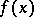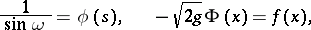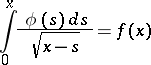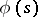# Abel problem

(diff) ← Older revision | Latest revision (diff) | Newer revision → (diff)
Jump to: navigation, search

To find, in a vertical plane, a curve such that a material point moving along it under gravity from rest, starting from a point with ordinate, will meet the-axis after a time, where the functionis given in advance. The problem was posed by N.H. Abel in 1823, and its solution involves one of the first integral equations — the Abel integral equation — which was also solved. In fact, ifis the angle formed by the tangent of the curve being sought with the-axis, thenIntegrating this equation betweenandand puttingone obtains the integral equationfor the unknown function, the determination of which makes it possible to find the equation of the curve being sought. The solution of the equation introduced above is: